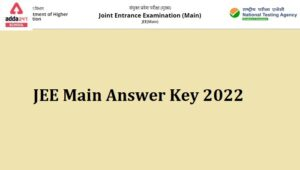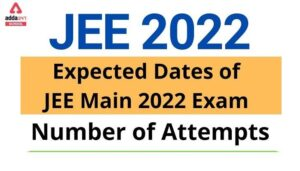Online Tution   »   JEE Main 2022: Mathematics Study Tips,...

# JEE Main 2022: Maths Study Tips and Important Topics

## JEE Main 2022 Maths

Mathematics is considered to be the most challenging subject in JEE Main 2022. Some students like maths problems and some students have fear of solving mathematical problems. To make preparation easy for JEE aspirants we have come up with this article. This article will assist JEE Aspirants to prepare for Mathematics. In Mathematics, students need practice and proper planning and execution. One can easily score good marks in JEE Main 2022 Mathematics by solving problems and giving mock tests. Through mock tests, the students can assess their preparation and also mock tests help students to find their weak areas. With the help of mock tests, students can get a variety of questions and they can consolidate their weak areas by figuring out them. In this article, we have discussed the tips and tricks, Maths pattern, maths weightage in JEE Main 2022. The students preparing for JEE Main 2022 and who wish to appear in JEE Main can bookmark this page to get all the latest updates regarding JEE Main 2022.

## JEE Main 2022 Maths Exam Tips

Mathematics plays a vital role in JEE Main 2022. Hence, students have to score good marks in Mathematics. A total of 30 questions will be asked from mathematics in JEE Main 2022. Below we have provided a brief detail about mathematics in JEE Main 2022.

 Mode of Examination Online Total Number of Questions in Mathematics 30 Total Number of Questions to be Attempted 25 Type of Questions MCQ and Non-MCQs Marking Scheme Correct Answer: +4 Negative Marking Incorrect Answer: -1 & No Negative Marking for Non-MCQs Medium of Paper English, Hindi, Gujarati## JEE Main 2022 Maths Important Topics Weightage

The weighting of the topics is listed in the table below, based on the previous year’s patterns. However, depending on the syllabus and exam pattern, this weightage may change from year to year. For reference the students can consider it and can start their preparation according to the maths weightage given below in the table:

 Topics No of Questions Weightage of Marks Matrices and Determinants 2 8 Vector Algebra 2 8 Three Dimensional Geometry 2 8 Complex numbers and Quadratic Equation 2 8 Limits, Continuity, and Differentiability 3 12 Integral Calculus 3 12 Coordinate Geometry 5 20 Trigonometry 1 4 Statics and Dynamics 1 4 Statistics and Probability 2 8 Sets, Relation, and Function 1 4 Sequences and Series 1 4 Binomial Theorem and Its Application 1 4 Differential Equation 1 4 Differential Calculus 1 4 Permutations and Combinations 1 4 Mathematical Reasoning 1 4 Total 30 120

Read More: JEE Main Application Form 2022: Dates, Fee, direct link at jeemain.nta.nic.in

## JEE Main 2022 Maths Important Topics

Algebra: There are only a few formulae in this section, mostly properties. Be clear with how the symmetric sums of roots occur in an equation as well as the transformation of equations in Quadratic or Theory of Equations. In Complex Numbers, it is critical to review the geometric meaning of many formulae; this makes them more intuitive and easier to recall. Binomial, permutation and combination, and probability are all intertwined and should be reviewed as a whole. It’s also a good idea to identify a few common problems to revise in the latter two because they’re less formula-based and more targeted toward problem-solving tactics.

Calculus: The standard limits, differentiation equations, and standard integration findings should all be extremely clear to the candidates. In addition, review the theoretical prerequisites for Continuity and Differentiability, as well as Definite Integration characteristics.

Co-ordinate Geometry: Examine the formulae for any similarities between them, as this will aid remember during the exam. Geometrical properties, particularly for straight lines and circles, should not be overlooked and should be included in your notes.

Vector and 3-D Geometry: Because they are so closely related, try to do them at the same time. Standard equations are likely to be used in this section’s questions.

Trigonometry: Divide your notes into four sections: fundamental formulae, trigonometric equations, inverses, and triangle solutions. In truth, there appear to be a lot of formulas in this chapter of JEE. Keep in mind that the main questions will most likely be based on the most prevalent outcomes.

## JEE Main 2022 Maths Exam Marking Scheme

Here we have provided the marking scheme of JEE Main 2022. A total of 90 questions will be asked and each subject holds a maximum of 100 marks. Questions will be asked from Maths, Physics, and chemistry. Below we have given the marking scheme table for JEE aspirants.

 Subjects Maximum Questions Maximum Marks Physics 30 100 Chemistry 30 100 Mathematics 30 100 Total 90 questions 300 marks

#### Per Question Marking Scheme

Students will be awarded 4 marks if they mark the right answer and if they mark the wrong answer then 1 mark will be deducted. The negative marking is applicable only for multiple-choice questions. There is no negative marking for Numeric value type questions. Check the per question marks break-up given below in the table:

 Type of question Negative marking Marks Per Right Answer MCQs -1 Mark +4 Marks Numeric Value Questions No negative marking +4 Marks

Read More: Complete Study Plan And Preparation Tips To Crack JEE Main 2022

## FAQs on JEE Main 2022: Maths Study Tips and Important Topics

Q. Is there any negative marking in JEE Main 2022?

Yes, there is a negative marking of ¼ in JEE Main 2022 for Multiple choice questions and there is no negative marking for Numeric Value questions.

Q. What is the marking scheme of JEE Main 2022?

Four marks will be awarded for each correct answer and 1 mark will be deducted for each wrong multiple choice answer there is no negative marking for numeric value questions.

Q. How many questions will be asked from Maths in JEE Main 2022?

There will be a total of 30 questions asked from Maths in JEE Main 2022.

Q. How many questions will be asked from physics in JEE Main 2022?

There will be a total of 30 questions asked from physics in JEE Main 2022.

Q. How many questions will be asked from Chemistry in JEE Main 2022?

There will be a total of 30 questions asked from Chemistry in JEE Main 2022.

Sharing is caring!

## FAQs

### Q. Is there any negative marking in JEE Main 2022?

Yes, there is a negative marking of ¼ in JEE Main 2022 for Multiple choice questions and there is no negative marking for Numeric Value questions.

### Q. What is the marking scheme of JEE Main 2022?

Four marks will be awarded for each correct answer and 1 mark will be deducted for each wrong multiple choice answer there is no negative marking for numeric value questions.

### Q. How many questions will be asked from Maths in JEE Main 2022?

There will be a total of 30 questions asked from Maths in JEE Main 2022.

### Q. How many questions will be asked from physics in JEE Main 2022?

There will be a total of 30 questions asked from physics in JEE Main 2022.

### Q. How many questions will be asked from Chemistry in JEE Main 2022?

There will be a total of 30 questions asked from Chemistry in JEE Main 2022.

### TOPICS:

•JEE Main Answer Key 2022- Release Date &...
•JEE Main 2022 All India Live Free Mock T...
•How Will Having Only 2 Attempts For JEE...
•JEE Main 2022 Attempts and Dates: Studen...
•How to do Preparation for JEE Main 2022 ...
•JEE Main Application Form 2022